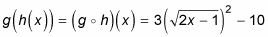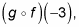##### Music Composition For DummiesA composition of functions is one function acting upon another. Think of it like putting one function inside of the other — f(g(x)), for instance, means that you plug the entire g(x) function in for all x’s in f(x). To solve such a problem, you work from the inside out:

f(g(x)) = f(3x2 – 10) = (3x2 – 10)2 – 6(3x2 – 10) + 1

This process puts the g(x) function into the f(x) function everywhere the f(x) function asks for x. This equation ultimately simplifies to 9x4 – 78x2 + 161, in case you’re asked to simplify the composition (which you usually are).

Likewise,which easily simplifies to 3(2x – 1) – 10 because the square root and square cancel each other. This equation simplifies even further to 6x – 13.

You may also be asked to find one value of a composed function. To findfor instance, it helps to realize that it’s like reading Hebrew: You work from right to left. In this example, you’re asked to put –3 in for x in f(x), get an answer, and then plug that answer in for x in g(x). Here are these two steps in action:

f(–3) = (–3)2 – 6(–3) + 1 = 28

g(28) = 3(28)2 – 10 = 2,342